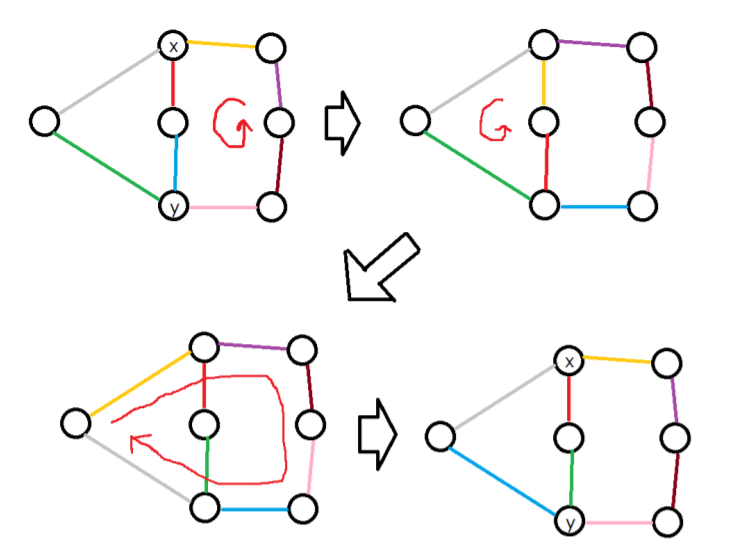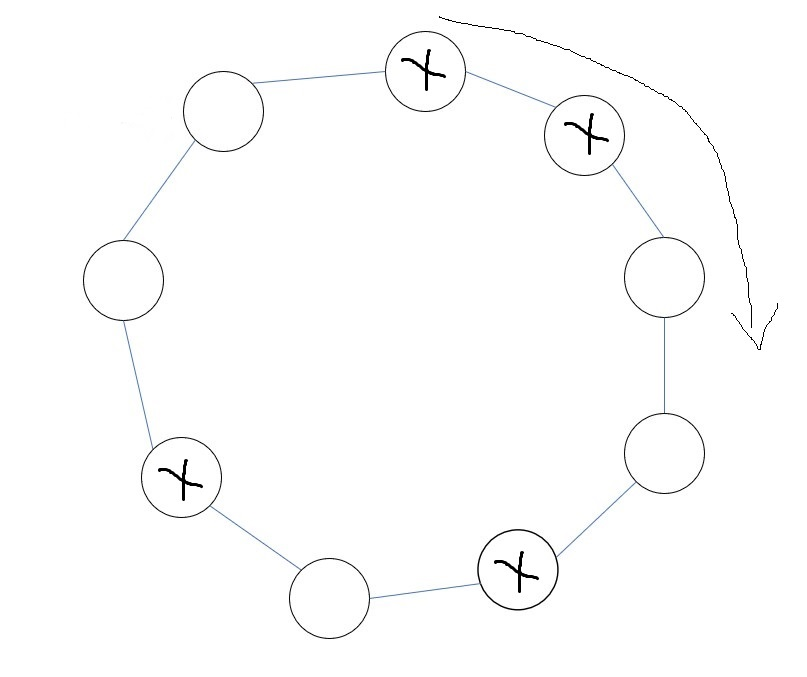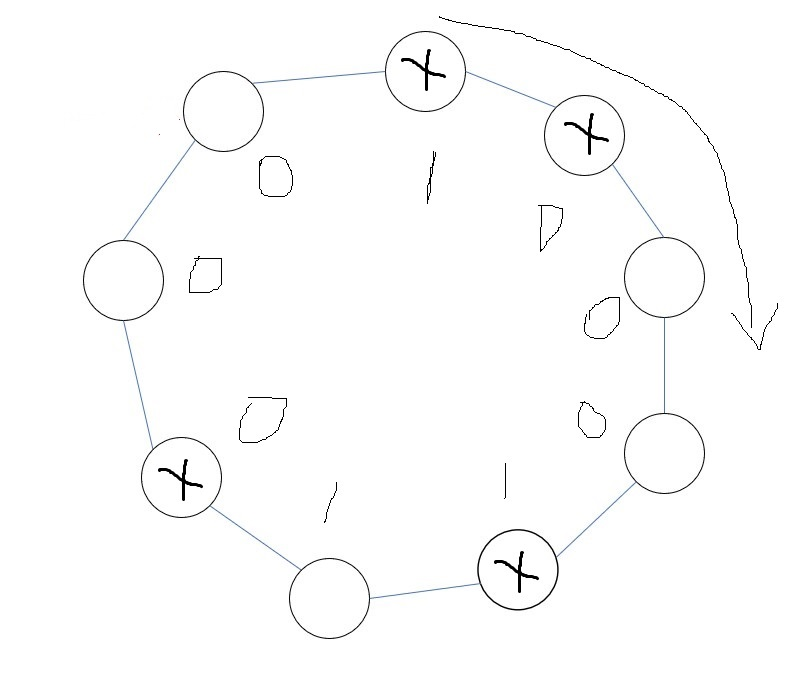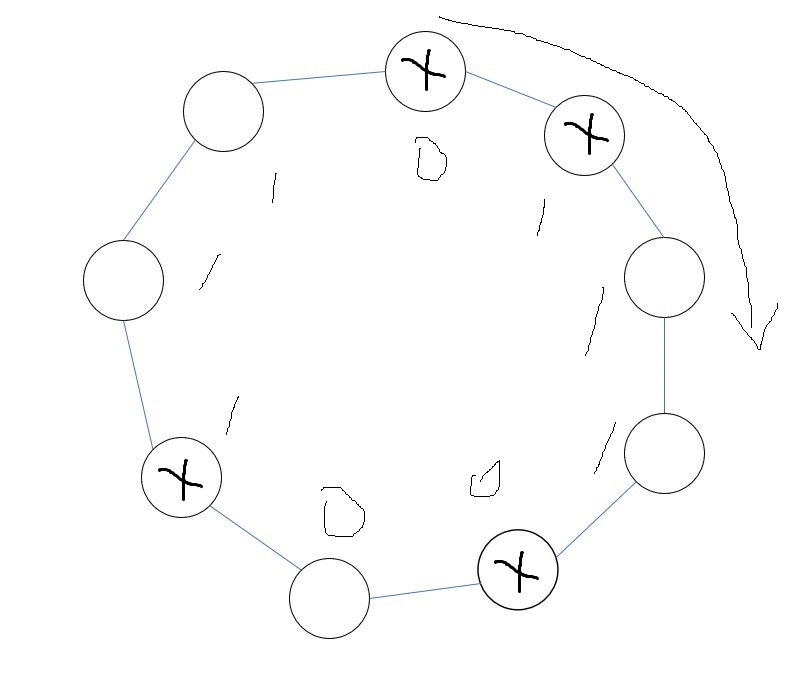# 组合计数练习题

## UOJ422 小z的礼物

$\max (S) = \sum_{T\subseteq S} (-1)^{|T|+1} \min (T)$

$f_{S,t}$表示上一列选了的格子的集合是$S$，之前选过的格子的（子集内每一个物品包含它的相邻格子对数量的和）-（同时包含了子集内的两个物品的相邻格子对的数量）为$t$，所有方案的容斥系数的和。用类似轮廓线dp的方法转移，就可以做到$O(2^n n^2m^2)$的复杂度。

Code

## LOJ2320「清华集训 2017」生成树计数

$val (T) = (\sum_{i=1}^n d_i^m) (\prod_{i=1}^n d_i^m )(\prod_{i=1}^n a_i^{d_i})$

$Ans = \sum_{k_1+k_2+\cdots +k_n=n-2} {(n-2)!\over k_1!k_2!\cdots k_n!} \cdot \sum_{i=1}^n (k_i+1)^{2m}a_i^{k_i+1} \prod_{j\neq i} (k_j+1)^ma_j^{k_j+1}$

$A_i(x) = \sum_{k=0}^\infty {a_i^{k+1}(k+1)^m\over k!} x^k\\ B_i(x) = \sum_{k=0}^\infty {a_i^{k+1}(k+1)^{2m} \over k!}x^k$

$Ans= (n-2)!\sum_{i=1}^n (B_i(x)\prod_{j\neq i} A_j(x) )[x^{n-2}]$

$T(x) = \sum_{k=0}^\infty {a_i^{k+1}(k+1)^m \over (k+1)!} x^{k+1}\\ =\sum_{k=1}^\infty {a_i^kk^m\over k!}x^k$

$k^m$展开：

$T(x) = \sum_{k=1}^\infty {a_i^k \over k!} x^k \sum_{j=0}^{\min\{m,k\}} \begin{Bmatrix}m\\ j\end{Bmatrix} {k\choose j} j!$

${k\choose j}j!$与前面的$k!$抵消，得到

$T(x) = \sum_{k=1}^\infty a_i^k x^k \sum_{j=0}^{\min\{m,k\}} {1\over (k-j)!}\begin{Bmatrix}m\\ j\end{Bmatrix}$

$j$提前

$T(x) = \sum_{j=0}^m \begin{Bmatrix}m\\ j\end{Bmatrix} \sum_{k=j}^\infty {a_ix^k\over (k-j)!}\\ =\sum_{j=0}^m \begin{Bmatrix}m\\ j\end{Bmatrix} a_i^jx^j \sum_{k=0}^\infty {a_i^kx^k\over k!}\\ =\sum_{j=0}^m \begin{Bmatrix}m\\ j\end{Bmatrix} a_i^jx^j e^{a_ix}$

$A_i(x) = T'(x)\\ =\sum_{j=0}^m \begin{Bmatrix}m\\ j\end{Bmatrix} (j \cdot a_i^jx^{j-1} e^{a_ix} + a_i \cdot a_i^jx^j e^{a_ix}) \\ =\sum_{j=0}^{m} \begin{Bmatrix}m\\ j+1\end{Bmatrix} (j+1)( a_i^{j+1}x^je^{a_ix}) + \sum_{j=0}^{m} \begin{Bmatrix}m\\ j\end{Bmatrix} (a_i^{j+1} x^j e^{a_ix}) \\ = \sum_{j=0}^{m}(\begin{Bmatrix}m\\ j+1\end{Bmatrix} (j+1)+\begin{Bmatrix}m\\ j\end{Bmatrix} ) a_i^{j+1}x^je^{a_ix}\\ = e^{a_ix}\sum_{j=0}^{m}\begin{Bmatrix}m+1\\ j+1\end{Bmatrix} a_i^{j+1}x^j\\$

$B_i(x)$同理，得到

$B_i(x) = e^{a_ix}\sum_{j=0}^{2m}\begin{Bmatrix}2m+1\\ j+1\end{Bmatrix} a_i^{j+1}x^j\\$

$\sum_{i=1}^n B_i(x)\prod_{j\neq i} A_i(x)\\ = e^s \sum_{i=1}^n (\sum_{j=0}^{2m}\begin{Bmatrix}2m+1\\ j+1\end{Bmatrix} a_i^{j+1}x^j) \prod_{k\neq i}(\sum_{j=0}^{m}\begin{Bmatrix}m+1\\ j+1\end{Bmatrix} a_k^{j+1}x^j)\\$

Code

## ARC062D Painting Graphs with AtCoDeerCode

## HDU6402 Boolean 3-Array$\sum_{x\in a'} a_x \operatorname{lcm}{\{la,lb,lc\}\over la} +\sum_{x\in b'} b_x \operatorname{lcm}{\{la,lb,lc\}\over lb} + \sum_{x\in c'} c_x {\operatorname{lcm}\{la,lb,lc\}\over lc} \equiv 0 \pmod 2$

#include <cstdio>
#include <iostream>
#include <cstring>
#include <algorithm>
#include <vector>
#include <bitset>
#define PB push_back
#define MP make_pair
#define PII pair<int,int>
#define FIR first
#define SEC second
#define ll long long
using namespace std;
template <class T>
inline void rd(T &x) {
x=0; char c=getchar(); int f=1;
while(!isdigit(c)) { if(c=='-') f=-1; c=getchar(); }
while(isdigit(c)) x=x*10-'0'+c,c=getchar(); x*=f;
}
const int mod=998244353;
int Pow(int x,int y) {
int res=1;
for(;y;x=x*(ll)x%mod,y>>=1)
if(y&1) res=res*(ll)x%mod;
return res;
}
int lcm;
int fac,inv,ipw,pw;
int Inv;
vector<vector<PII> > g;
namespace Mat {
bitset<39> f,b;
int m,cnt;
void init(int len) { for(int i=0;i<m;++i) f[i].reset(); m=len,cnt=0; }
void ins(int l,int r,int x) {
if(x&1) for(int i=l;i<r;++i) b[i]=1;
}
void Ins() {
// cout<<"INS:"; for(int i=0;i<m;++i) cout<<(b[i]==1); cout<<endl;
for(int i=0;i<m;++i) if(b[i]) {
if(f[i][i]) b^=f[i];
else {
f[i]=b,cnt++;
break;
}
}
b.reset();
}
int sol() { return cnt; }
void Debug() {
for(int i=0;i<m;++i,puts(""))
for(int j=0;j<m;++j) cout<<(f[i][j]==1);
}
}
namespace predo_calc {
int gcd(int a,int b) { return b?gcd(b,a%b):a; }
int LCM(int a,int b) { return a/gcd(a,b)*b; }
vector<PII> s;
int cur;
void dfs(int u,int tot) {
if(tot==cur) { g[cur].PB(s); return; }
if(tot+u>cur) return;
dfs(u+1,tot);
for(int j=1;tot+j*u<=cur;++j) {
s.PB(MP(u,j));
dfs(u+1,tot+j*u);
s.pop_back();
}
}
void getpw(int n) {
pw=1; for(int i=1;i<=n;++i) pw[i]=pw[i-1]*2ll%mod;
ipw[n]=Pow(pw[n],mod-2); for(int i=n;i>=1;--i) ipw[i-1]=ipw[i]*2ll%mod;
}
void predo(int n) {
for(int i=1;i<=n;++i) Inv[i]=Pow(i,mod-2);
fac=1; for(int i=1;i<=n;++i) fac[i]=fac[i-1]*(ll)i%mod;
inv[n]=Pow(fac[n],mod-2); for(int i=n;i>=1;--i) inv[i-1]=inv[i]*(ll)i%mod;
getpw(n*n*n);
for(int i=1;i<=n;++i)
for(int j=1;j<=n;++j)
for(int k=1;k<=n;++k)
lcm[i][j][k]=LCM(LCM(i,j),k);
for(int i=1;i<=n;++i) cur=i,dfs(1,0);
}
}
int sol(int A,int B,int C) {
int ans=0;
for(int pa=0;pa<g[A].size();++pa)
for(int pb=0;pb<g[B].size();++pb)
for(int pc=0;pc<g[C].size();++pc) {
int tans=1;
vector<PII> &pA=g[A][pa],&pB=g[B][pb],&pC=g[C][pc];
for(int j=0;j<pA.size();++j) tans=tans*(ll)inv[pA[j].SEC]%mod*Pow(Inv[pA[j].FIR],pA[j].SEC)%mod;
for(int j=0;j<pB.size();++j) tans=tans*(ll)inv[pB[j].SEC]%mod*Pow(Inv[pB[j].FIR],pB[j].SEC)%mod;
for(int j=0;j<pC.size();++j) tans=tans*(ll)inv[pC[j].SEC]%mod*Pow(Inv[pC[j].FIR],pC[j].SEC)%mod;
Mat::init(A+B+C);
for(int iA=0,tA=0;iA<pA.size();++iA)
for(int jA=0;jA<pA[iA].SEC;++jA,tA+=pA[iA].FIR)
for(int iB=0,tB=A;iB<pB.size();++iB)
for(int jB=0;jB<pB[iB].SEC;++jB,tB+=pB[iB].FIR)
for(int iC=0,tC=A+B;iC<pC.size();++iC)
for(int jC=0;jC<pC[iC].SEC;++jC,tC+=pC[iC].FIR) {
int a=pA[iA].FIR,b=pB[iB].FIR,c=pC[iC].FIR;
tans=tans*(ll)pw[a*b*c/lcm[a][b][c]]%mod;
Mat::ins(tA,tA+pA[iA].FIR,lcm[a][b][c]/a);
Mat::ins(tB,tB+pB[iB].FIR,lcm[a][b][c]/b);
Mat::ins(tC,tC+pC[iC].FIR,lcm[a][b][c]/c);
Mat::Ins();
}
tans=tans*(ll)pw[A+B+C-Mat::sol()]%mod;
// Mat::Debug();
ans=(ans+tans)%mod;
}
ans=ans*(ll)ipw[A+B+C]%mod;
}

int main() {
predo_calc::predo(13);
int T; rd(T);
while(T--) {
int A,B,C; rd(A),rd(B),rd(C);
cout<<sol(A,B,C)<<endl;
}
return 0;
}


©️2019 CSDN 皮肤主题: 技术工厂 设计师: CSDN官方博客